The Depth-First Traversal or the DFS traversal of a Graph is used to traverse a graph depth wise. That is, it in this traversal method, we start traversing the graph from a node and keep on going in the same direction as far as possible. When no nodes are left to be traversed along the current path, backtrack to find a new possible path and repeat this process until all of the nodes are visited.

We can implement the DFS traversal algorithm using a recursive approach. While performing the DFS traversal the graph may contain a cycle and the same node can be visited again, so in order to avoid this we can keep track of visited array using an auxiliary array. On each step of the recursion mark, the current vertex visited and call the recursive function again for all the adjacent vertices.

Illustration:

Step 1: Consider the below graph and apply the DFS algorithm recursively for every node using an auxiliary stack for recursive calls and an array to keep track of visited vertices.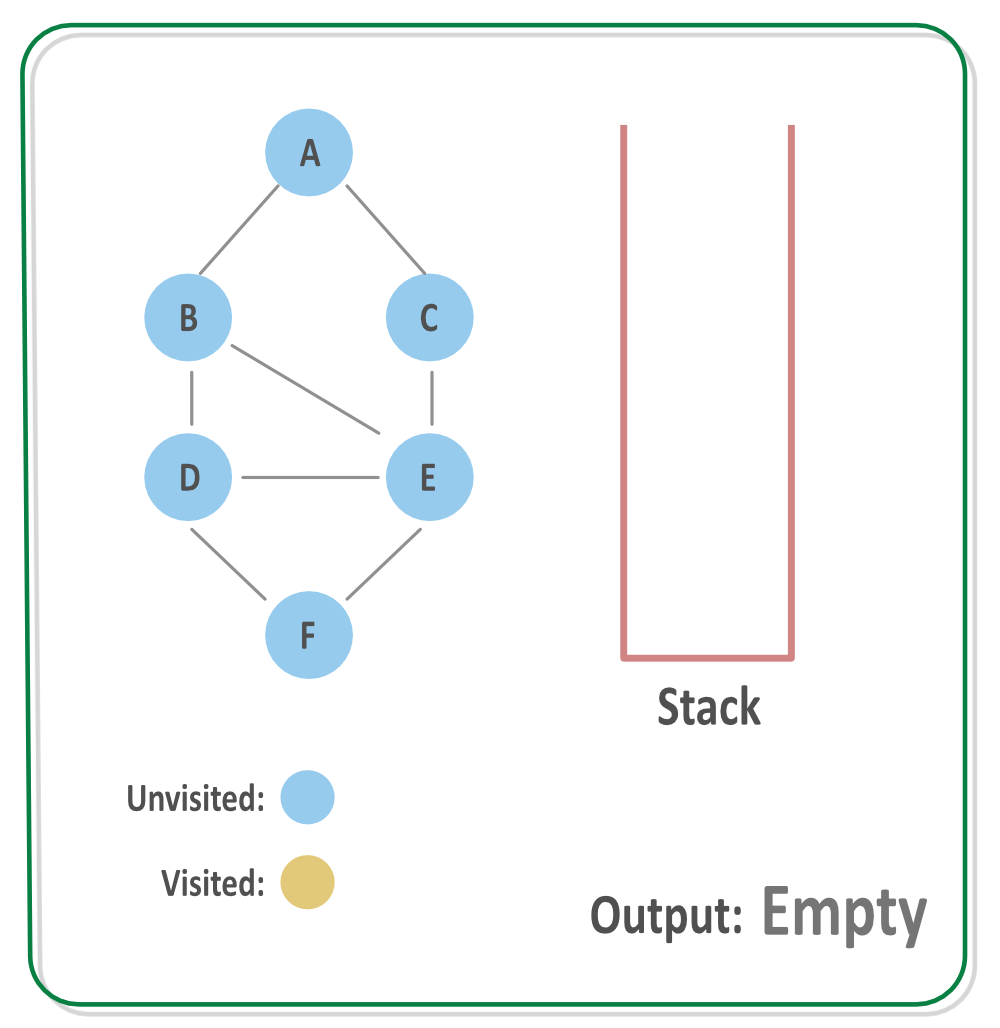Step 2: Process the vertex A, mark it visited and call DFS for its adjacent vertices. Suppose the first adjacent vertex processed is B. The vertex A is put on the auxilary stack for now.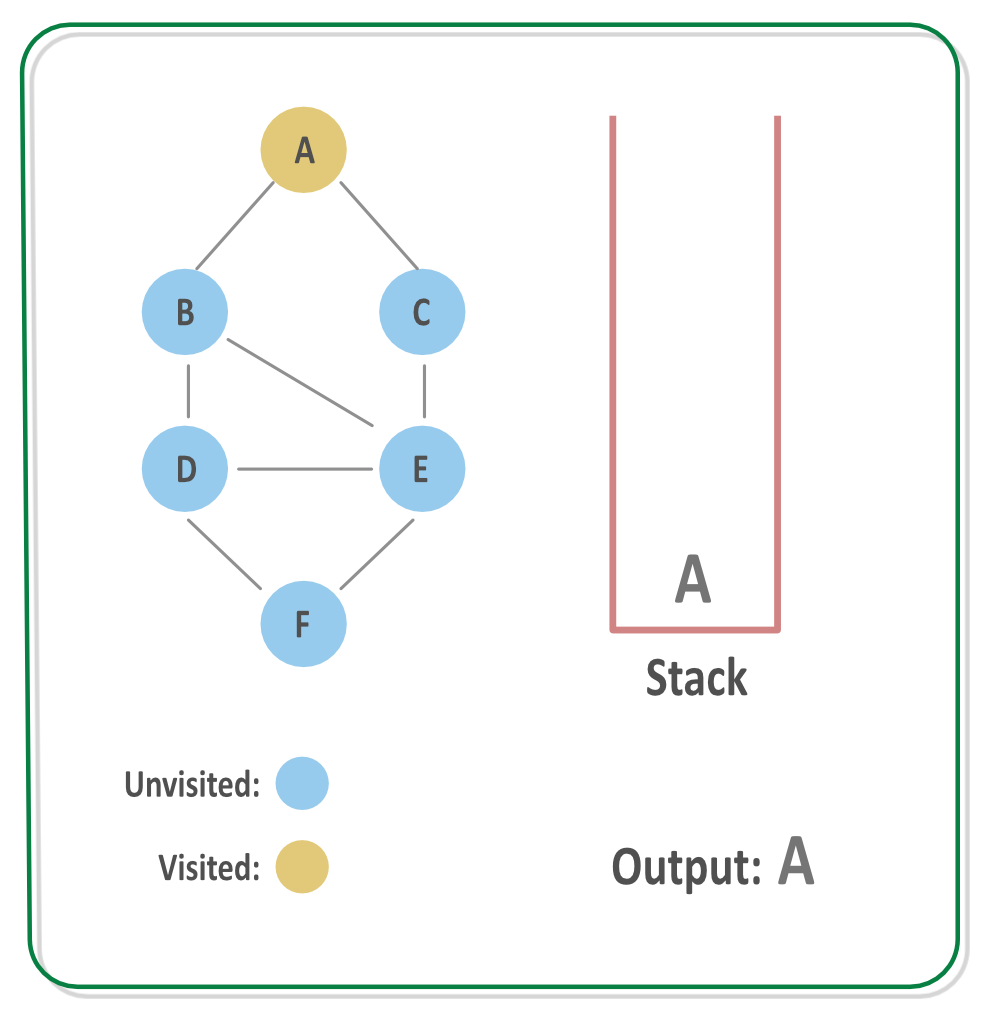Step 3: Process the vertex B, mark it visited and call DFS for its adjacent vertices. Suppose the first adjacent vertex processed is D. The vertex B is put on the auxilary stack for now.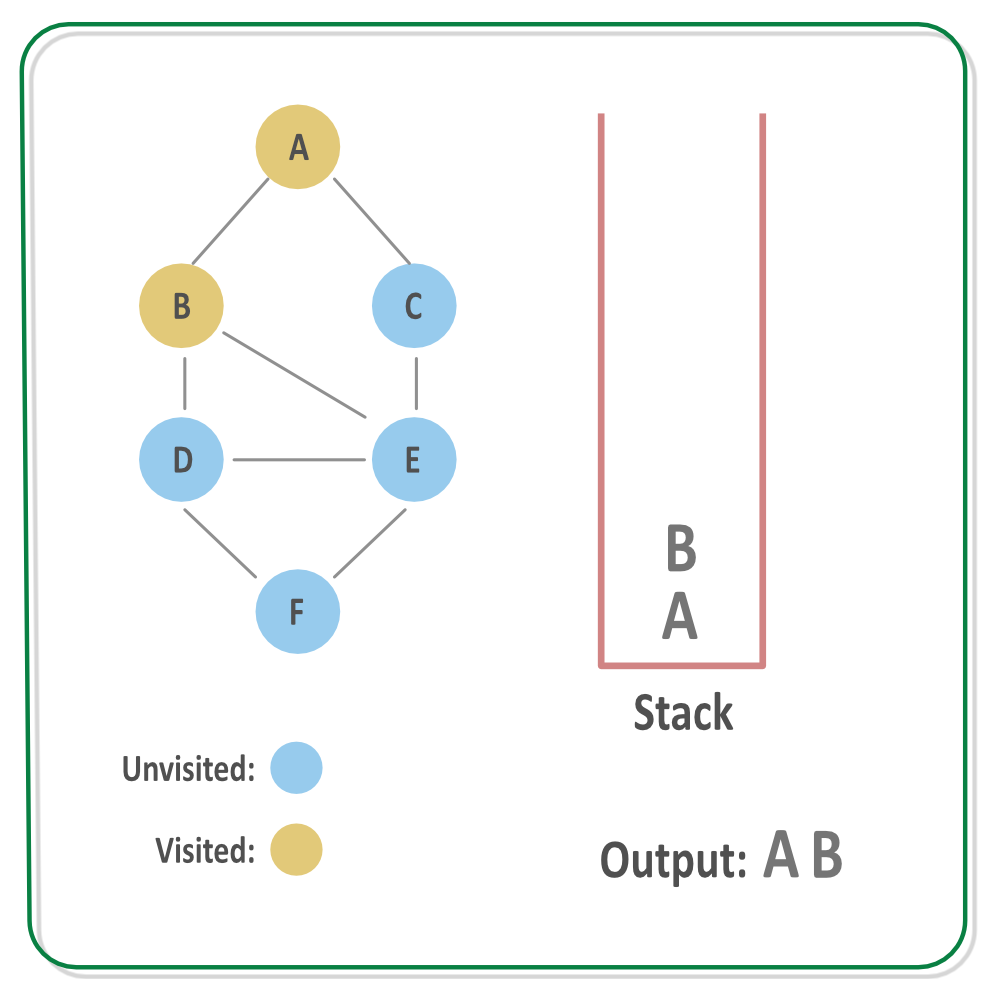Step 4: Process the vertex D, mark it visited and call DFS for its adjacent vertices. Suppose the first adjacent vertex processed is E. The vertex D is put on the auxilary stack for now.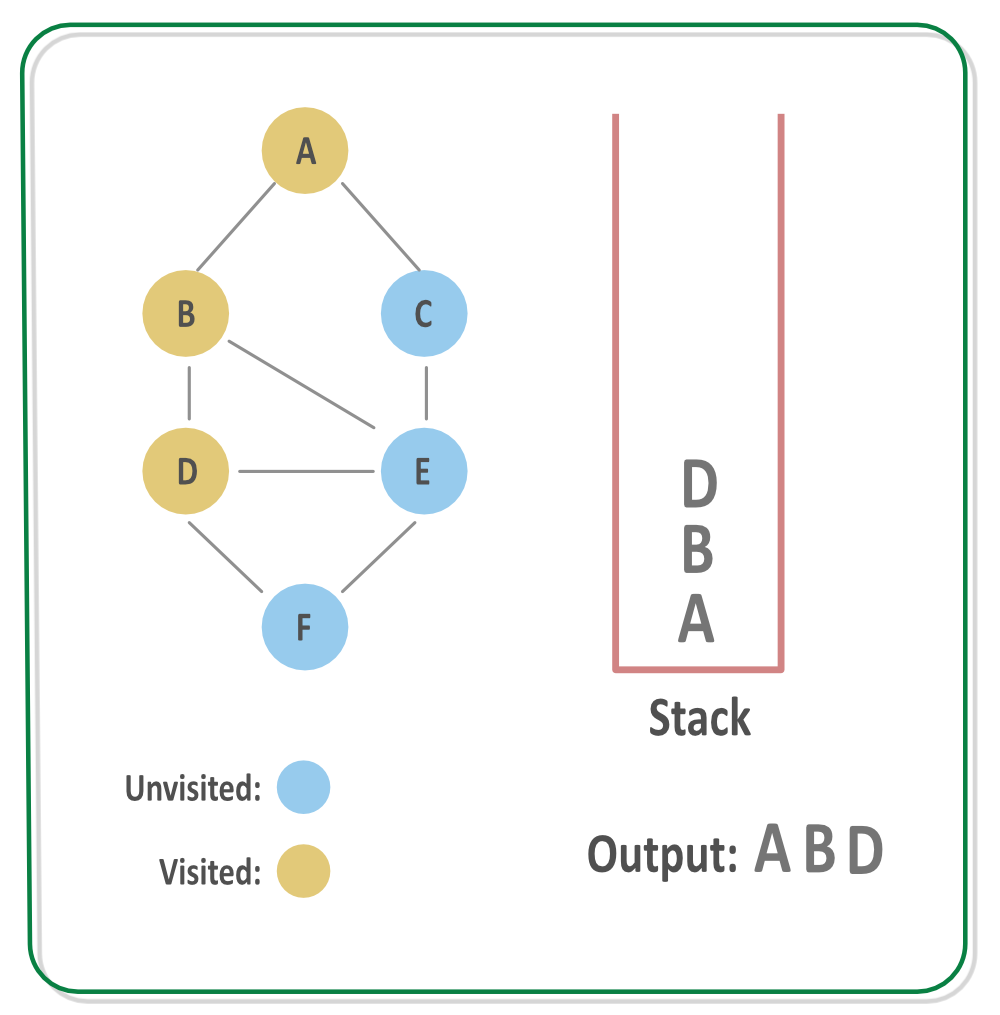Step 5: Process the vertex E, mark it visited and call DFS for its adjacent vertices. Suppose the first adjacent vertex processed is F. The vertex E is put on the auxilary stack
for now.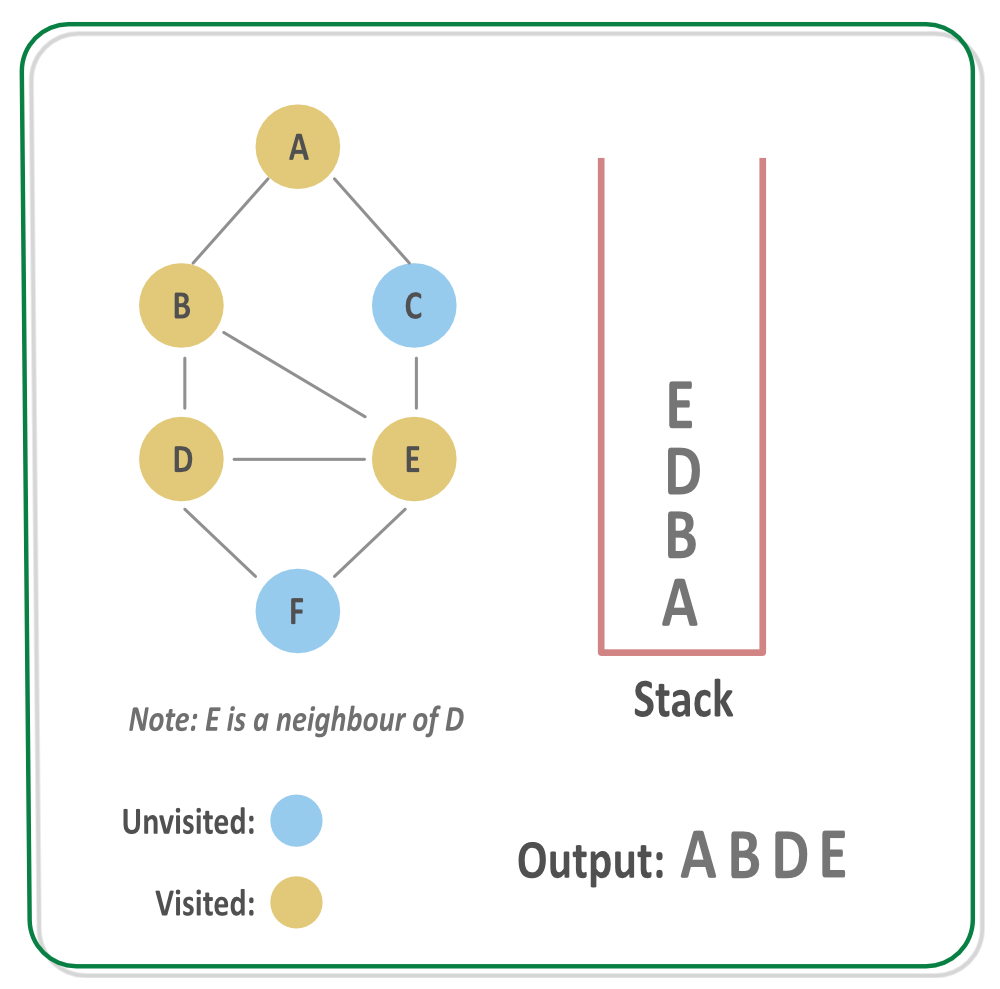Step 6: Process the vertex F, mark it visited and call DFS for its adjacent vertices. There are no adjacent vertex of the vertex F, so backtrack to find a new path. The vertex F is put on the auxilary stack for now.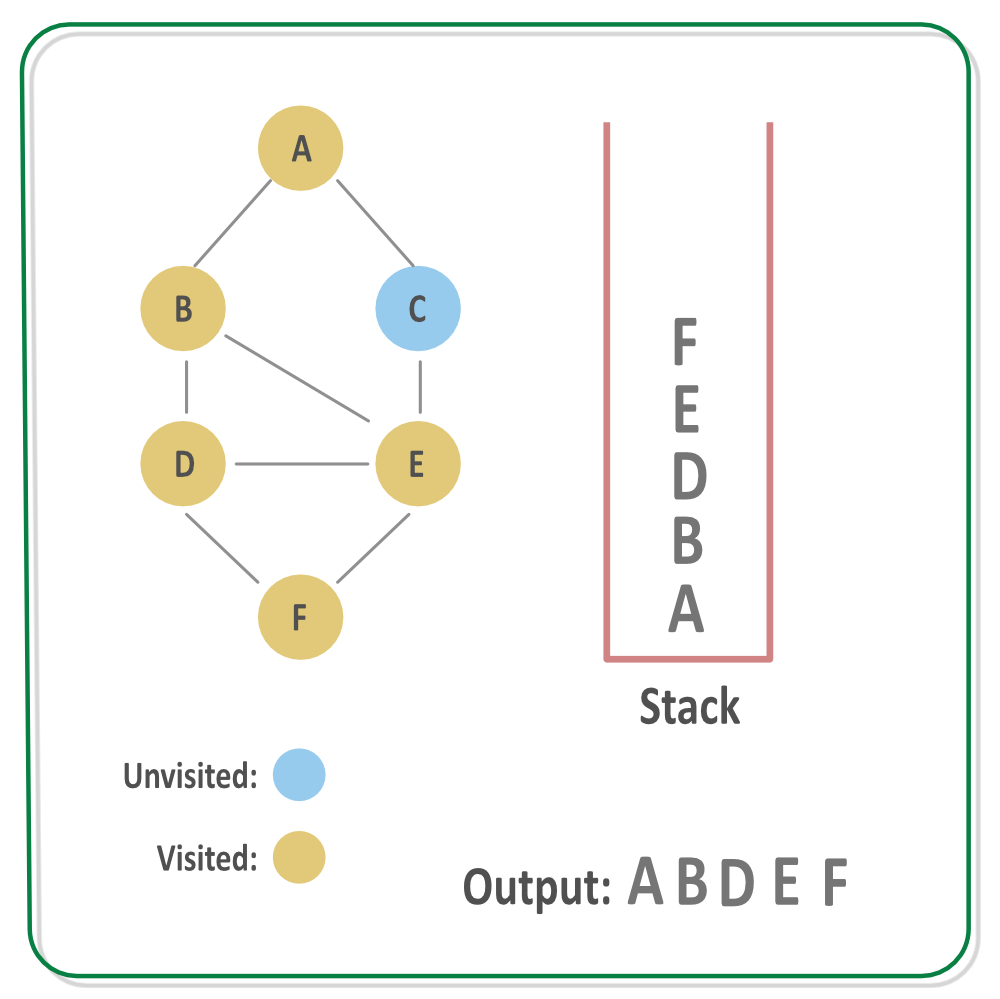Step 7: Since the vertex F has no adjacent vertices left unvisited, backtrack and go to previous call, that is process any other adjacent vertex of node E, that is C.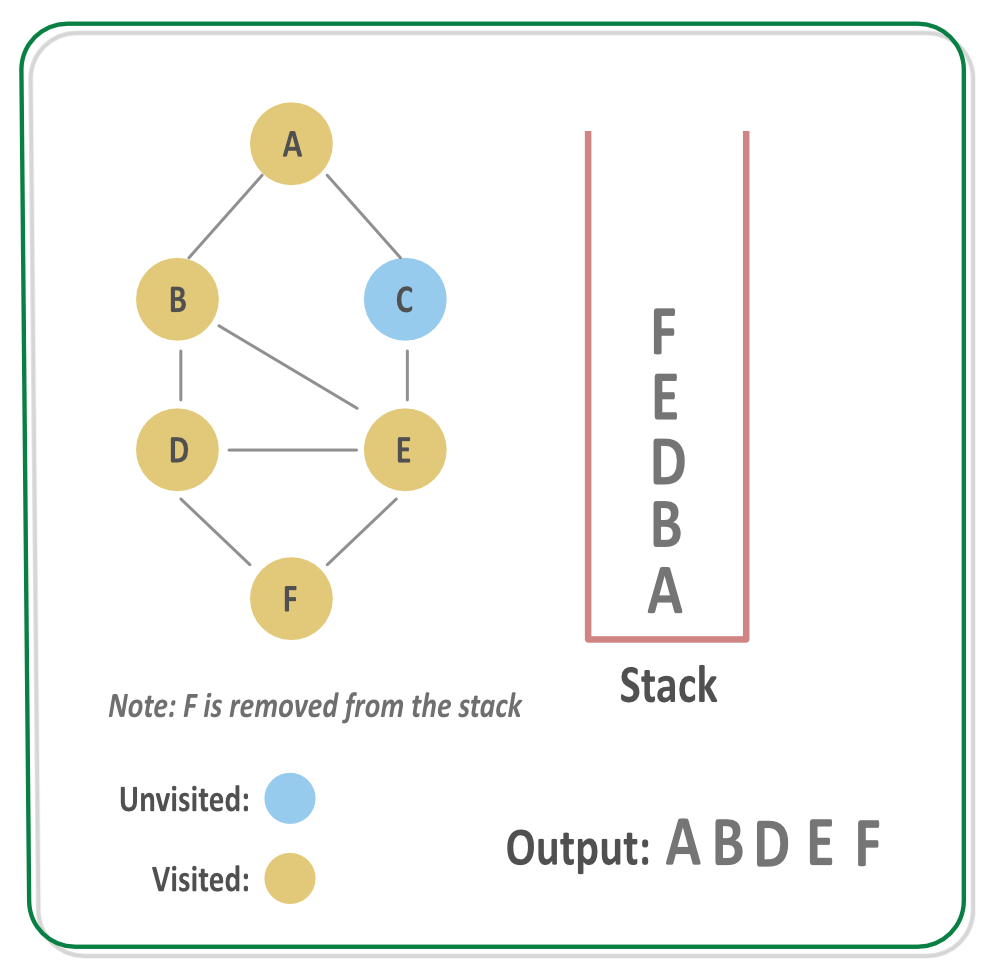Step 8: Process the vertex C, mark it visited and call DFS for its adjacent vertices. The vertex C is put on the auxilary stack for now.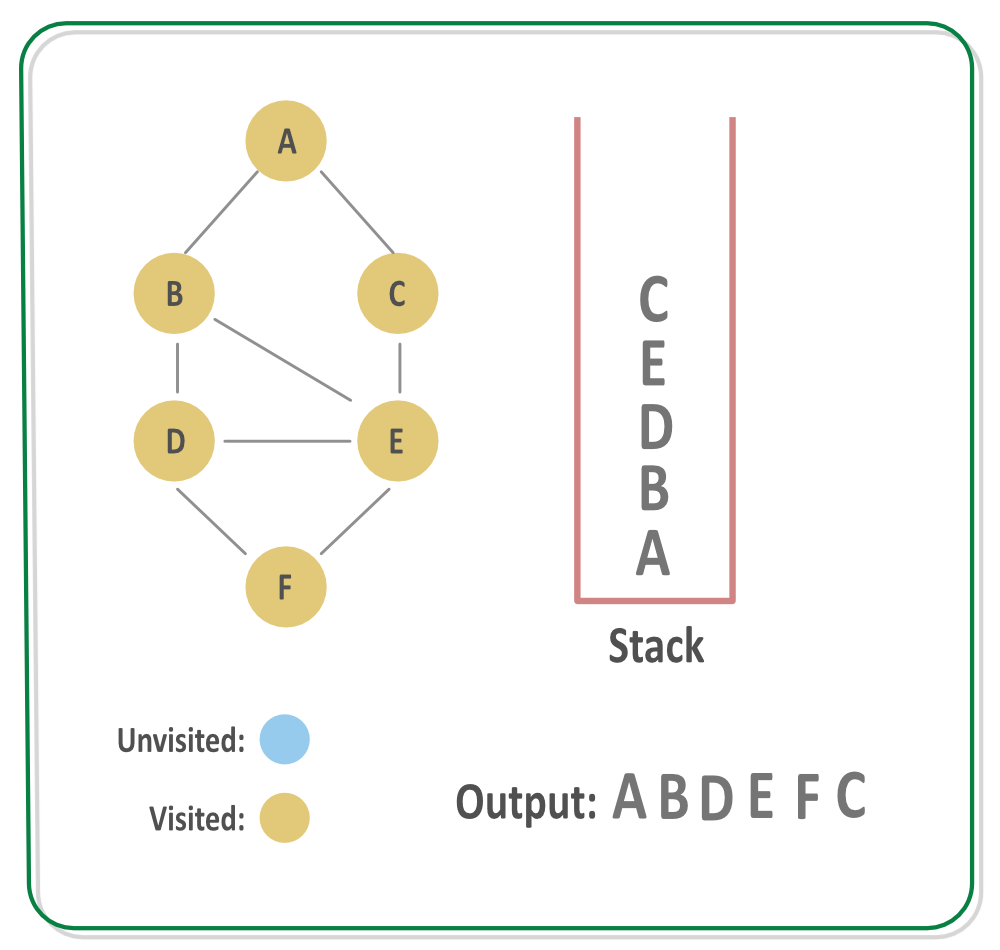Step 9: Since there are no adjacent vertex of C, backtrack to find a new path and keep removing nodes from stack until a new path is found. Since all of the nodes are processed so the stack will get empty.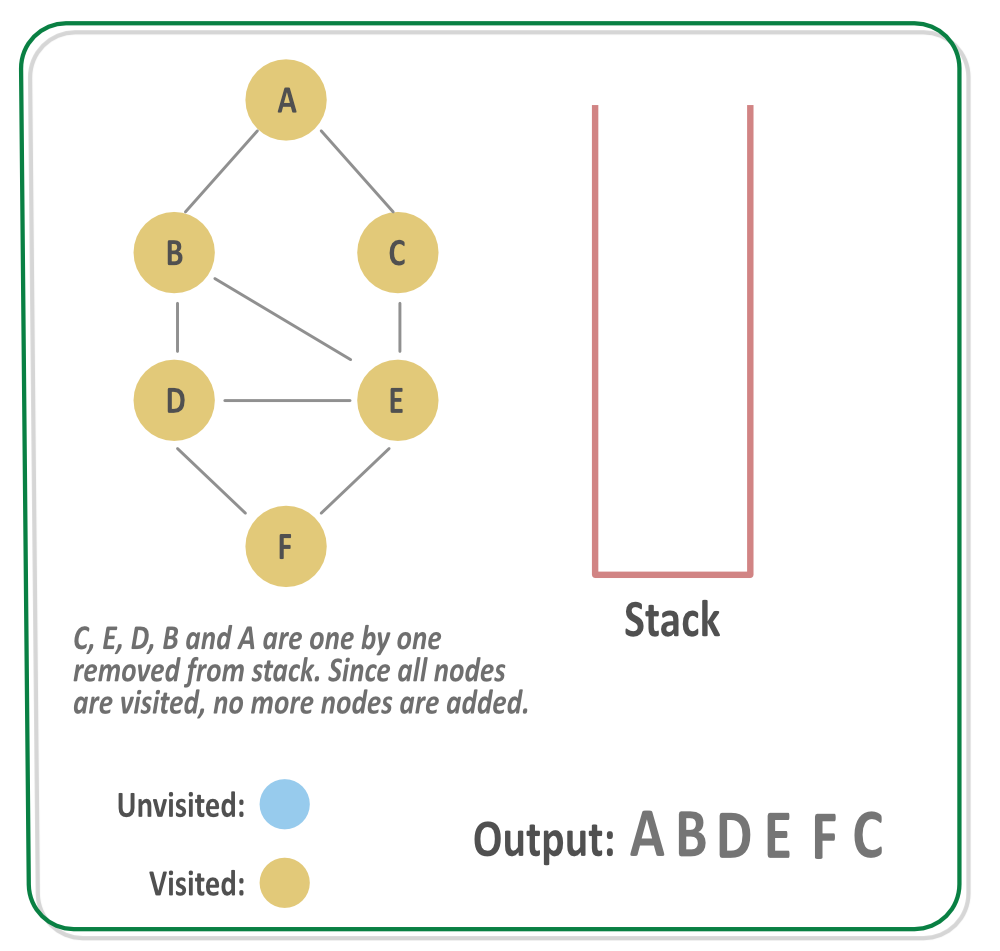c++:
```// C++ program to print DFS traversal from
// a given vertex in a  given graph
#include<iostream>
#include<list>
using namespace std;

// Graph class represents a directed graph
class Graph
{
int V;    // No. of vertices

// Pointer to an array containing

// A recursive function used by DFS
void DFSUtil(int v, bool visited[]);
public:
Graph(int V);   // Constructor

// function to add an edge to graph

// DFS traversal of the vertices
// reachable from v
void DFS(int v);
};

Graph::Graph(int V)
{
this->V = V;
}

{
}

void Graph::DFSUtil(int v, bool visited[])
{
// Mark the current node as visited and
// print it
visited[v] = true;
cout << v << " ";

// Recur for all the vertices adjacent
// to this vertex
list<int>::iterator i;
if (!visited[*i])
DFSUtil(*i, visited);
}

// DFS traversal of the vertices reachable from v.
// It uses recursive DFSUtil()
void Graph::DFS(int v)
{
// Mark all the vertices as not visited
bool *visited = new bool[V];
for (int i = 0; i < V; i++)
visited[i] = false;

// Call the recursive helper function
// to print DFS traversal
DFSUtil(v, visited);
}

int main()
{
// Create a graph given in the above diagram
Graph g(4);

cout << "Following is Depth First Traversal"
" (starting from vertex 2) \n";
g.DFS(2);

return 0;
}```
```java:
// Java program to print DFS traversal from a given given graph
import java.io.*;
import java.util.*;

// This class represents a directed graph using adjacency list
// representation
class Graph
{
private int V;   // No. of vertices

// Array  of lists for Adjacency List Representation

// Constructor
Graph(int v)
{
V = v;
for (int i=0; i<v; ++i)
}

//Function to add an edge into the graph
{
}

// A function used by DFS
void DFSUtil(int v,boolean visited[])
{
// Mark the current node as visited and print it
visited[v] = true;
System.out.print(v+" ");

// Recur for all the vertices adjacent to this vertex
while (i.hasNext())
{
int n = i.next();
if (!visited[n])
DFSUtil(n, visited);
}
}

// The function to do DFS traversal. It uses recursive DFSUtil()
void DFS(int v)
{
// Mark all the vertices as not visited(set as
// false by default in java)
boolean visited[] = new boolean[V];

// Call the recursive helper function to print DFS traversal
DFSUtil(v, visited);
}

public static void main(String args[])
{
Graph g = new Graph(4);